# Patterns and Sequences Patterns and Sequences Patterns refer

• Slides: 9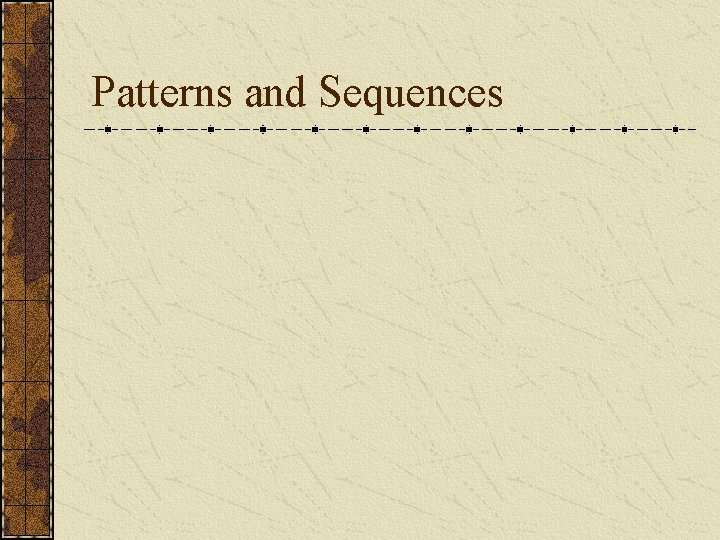Patterns and SequencesPatterns and Sequences Patterns refer to usual types of procedures or rules that can be followed. Patterns are useful to predict what came before or what might come after a set a numbers that are arranged in a particular order. This arrangement of numbers is called a sequence. For example: 3, 6, 9, 12 and 15 are numbers that form a pattern called a sequence The numbers that are in the sequence are called terms.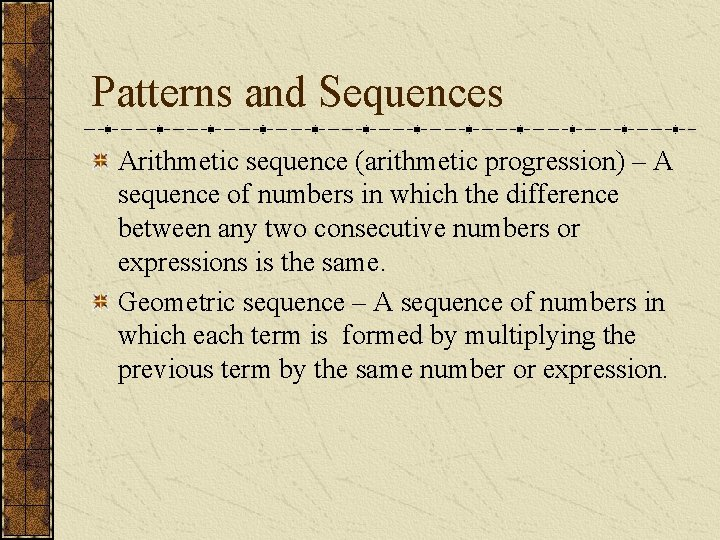Patterns and Sequences Arithmetic sequence (arithmetic progression) – A sequence of numbers in which the difference between any two consecutive numbers or expressions is the same. Geometric sequence – A sequence of numbers in which each term is formed by multiplying the previous term by the same number or expression.Arithmetic Sequence Find the next three numbers or terms in each pattern. Look for a pattern: usually a procedure or rule that uses the same number or expression each time to find the next term. The pattern is to add 5 to each term. The next three terms are: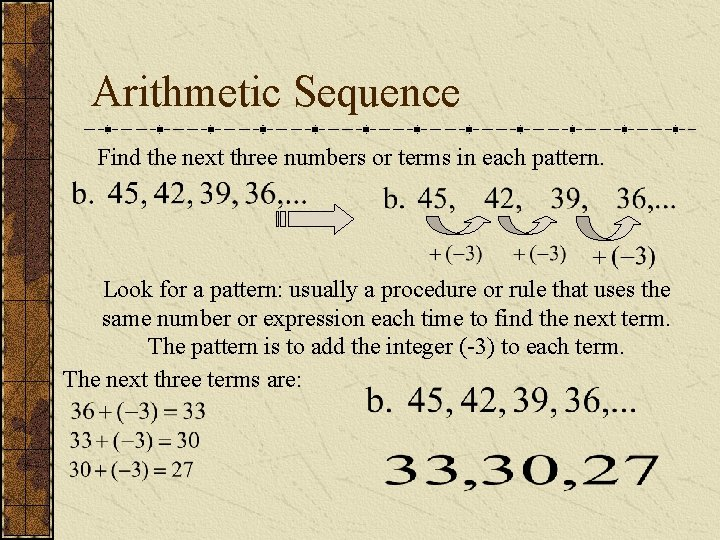Arithmetic Sequence Find the next three numbers or terms in each pattern. Look for a pattern: usually a procedure or rule that uses the same number or expression each time to find the next term. The pattern is to add the integer (-3) to each term. The next three terms are: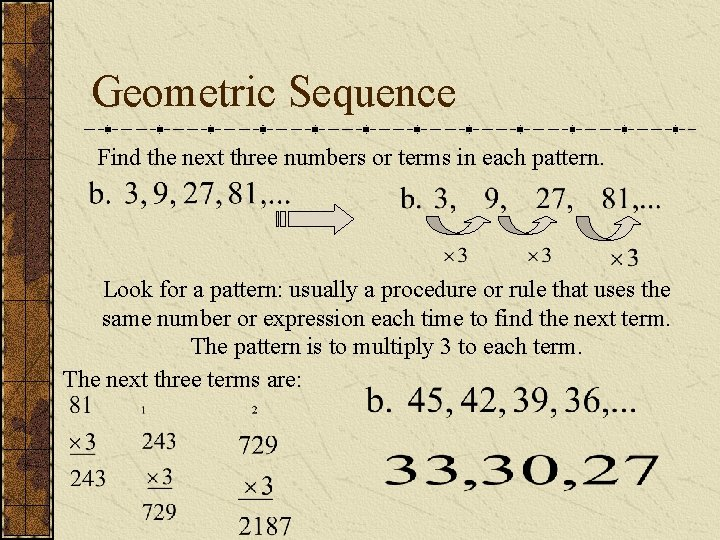Geometric Sequence Find the next three numbers or terms in each pattern. Look for a pattern: usually a procedure or rule that uses the same number or expression each time to find the next term. The pattern is to multiply 3 to each term. The next three terms are:Geometric Sequence Find the next three numbers or terms in each pattern. Look for a pattern: usually a procedure or rule that uses the same number or expression each time to find the next term. The pattern is to divide by 2 to each term. The next three terms are: Note: To divide by a number is the same as multiplying by its reciprocal. The pattern for a geometric sequence is represented as a multiplication pattern. For example: to divide by 2 is represented as the pattern multiply by ½.Geometric Sequence Find the next three expressions or terms in each pattern. Look for a pattern: usually a procedure or rule that uses the same number or expression each time to find the next term. The pattern is to multiply by 2 to each term or expression. The next three terms are: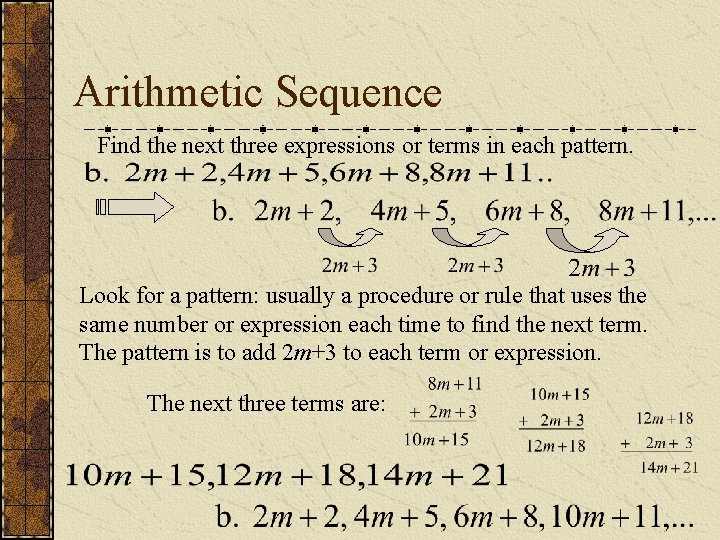Arithmetic Sequence Find the next three expressions or terms in each pattern. Look for a pattern: usually a procedure or rule that uses the same number or expression each time to find the next term. The pattern is to add 2 m+3 to each term or expression. The next three terms are: